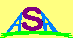### USEFUL APPROXIMATIONS FOR THE SOLAR POSITION

Some applications require a precise knowledge of the Sun's position, accurate to better than one minute of arc. A solar optical telescope that has to find and track the sun automatically is one example of such an application. However, other applications may need to know the solar position only to within a degree or two. These include most solar power systems (photovoltaic cells), and ionospheric models.A useful low resolution approximation of the Sun's declination (δ), longitude (λs) and local hour angle (HA) is given by the formulae:

δo = 23.5 sin ( YD - 82 )o

λso = 15 ( 12 - UT )

HAo = λs - λo

where
YD is the day of the year (1 to 365)
UT is the Universal Time in hours ( 0 to 23.9 )
λo is the observer's longitude

From these quantities the zenith angle (χ), elevation angle (el) and azimuth (Az) of the Sun may be calculated:

χ = cos-1 [ sin ( μo ) sin ( δ ) + cos ( μo ) cos ( δ ) cos ( HA ) ]
where
μo is the observer's latitude
Then:
elo = 90o - χ

Azo = cos-1 { [ sin ( δ ) - sin ( el ) sin ( μo ) ] / [ cos ( el ) cos ( μo ) ] }

where the azimuth angle needs to be adjusted to be in the same quadrant as the hour angle.

The errors from the above approximations are less than 2% in solar longitude and hour angle and less than 1% in solar declination. The conversions from equatorial coordinates (HA and δ) to horizon coordinates (azimuth and elevation) are exact.Australian Space Academy